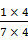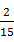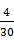### Sample Problem

Please order the fractions from least to greatest.

#### Solution==, multiply the numerator and the denominator of the fraction by the same number 4.==, multiply the numerator and the denominator of the fraction by the same number 2.

The same numerator, smaller denominators is with larger fraction.

So<<,<<Choice (b.) is correct.